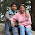## Monday, February 4, 2013

### Measurements Instrumentation Objective Questions: Part-4

 A hot-wire ammeter
A. Can measure ac as well as dc
B. Registers current changes very fast
C. Can indicate very low voltages
D. Measures electrical energy

 The device used to indicate the presence of an electric current is
A. Electrometer
B. Galvanometer
C. Voltmeter
D. Coulometer

 A watt-hour meter measures

A. Voltage
B. Current
C. Energy
D. Power

 A voltmeter should have
A. Low internal resistance
B. High internal resistance
C. Electrostatic plates
D. A sensitive amplifier

 An average-reading digital multimeter reads 10V when fed with a triangular wave, symmetric about the time-axis.For the same input an rms-reading meter will read  [GATE]
A. 20/√3
B. 10/√3
C. 20√3
D. 10√3

 The figure shows a three-phase delta connected load supplied from a 400V,50Hz,3-phase balanced source. The pressure coil(PC) and current coil(CC) of a wattmeter are connected to the load as shown,with the coil polarities suitably selected to ensure a positive deflection. The wattmeter reading will be   [GATE]
A. 0
B. 1600Watt
C. 800Watt
D. 400Watt

 The probes of a non-isolated, two channel oscilloscope are clipped to points A,B and C in the circuit of the adjacent figure. Vin is a square wave of a suitable low frequency. The display on Ch1 and Ch2 are as shown on the right. Then the "signal" and "ground" probes S1, G1 and S2, G2 of Ch1 and Ch2 respectively are connected to points [GATE 2007]

A.  A, B, C, A
B.  A, B, C, B
C.  C, B, A, B
D.  B, A, B, C

 An analog voltmeter uses external multiplier settings. With a multiplier setting of 20kΩ, it reads 440V and-with a multiplier setting of 80kΩ it reads 352V. For a multiplier setting of 40kΩ,voltmeter reads  [GATE2012]
A. 371V
B. 383V
C. 394V
D. 406V

 The rate at which charge carriers flow is measured in
A. Coulombs
B. Amperes
C. Watt-hour
D. Watts

 The main problem with bar-graph meters is that
A. They are not very sensitive
B. They are unstable
C. They cannot give very precise readings
D. They can display only peak values

 A dynamometer type wattmeter responds to the [Gate 1997]
(a) Average value of the active power
(b) Average value of reactive power
(c) Peak value of the active power
(d) Peak value of the reactive power

 A transfer instrument employed in the standardization of a polar type ac potentiometer is [Gate 1997]
(a) An electrostatic instrument
(b) A thermal instrument
(c) A dynamometer instrument
(d) A moving coil instrument

Please leave your comments below and subscribe to get the new posts directly to your mail...
8:03 PM

1.2.need problem answers with solution

3.thanks..........

4.Very useful ..:)
Thank u so much

5.6.7.very good